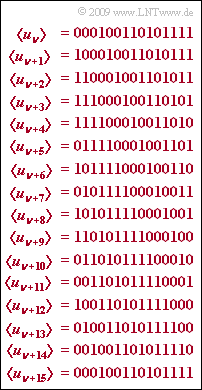# Exercise 5.3: PACF of PN SequencesM–sequence $(P = 15)$  plus cyclic permutations

With a feedback shift register of degree  $G$  a spreading sequence  $〈c_ν〉$  with the  (maximum)  period length  $P = 2^G - 1$  can be generated if the feedback coefficients  (taps)  are chosen correctly.

In this exercise,  we consider the PN generator with octal identifier  $(31)$  shown in the left graph of $\text{Example 1}$  in the theory section,  which yields a sequence with period length  $P = 15$  because of  $G = 4$.

The graph for this exercise shows the unipolar sequence  $〈u_ν〉$  with  $u_ν ∈ \{0, 1\}$  and cyclic shifts  $〈u_{ν+λ}〉$  derived from it,  where the shift parameter  $λ$  takes values between  $1$  and  $15$.   Here,  a shift by  $λ$  means absolutely an offset by  $λ · T_c$.  Here,   $T_c$  denotes the chip duration.

For use in a CDMA system,  however,  one uses the bipolar (antipodal) sequence  $〈c_ν〉$  with  $c_ν ∈ \{+1, -1\}$,  which is to be investigated starting in subtask  (5).   We are looking for its periodic auto-correlation function  $\rm (PACF)$

$${\it \varphi}_{c}(\lambda) = {\rm E} \big [ c_\nu \cdot c_{\nu+\lambda} \big ] \hspace{0.05cm}.$$

In order to derive it,  first the PACF

$${\it \varphi}_{u}(\lambda) = {\rm E}\big [ u_\nu \cdot u_{\nu+\lambda} \big ]$$

with the unipolar coefficients  $u_ν ∈ \{0, 1\}$,  will be calculated.  The conversion of the coefficients is given by  $c_ν = 1 - 2u_ν$.

Note:

### Questions

1

What is the degree of the PN generator?

 $G \ = \$

2

What is the expected squared value of the coefficients  $u_ν ∈ \{0,\ 1\}$?

 ${\rm E}\big[u_ν^{\hspace{0.04cm}2}\big] \ = \$

3

What is the expected squared value of the coefficients  $c_ν ∈ \{+1, –1\}$?

 ${\rm E}\big[c_ν^{\hspace{0.04cm}2}\big] \ = \$

4

Which statements are true for the expected value  ${\rm E}\big[u_ν · u_{ν+λ}\big]$?

 ${\rm E}\big[u_ν · u_{ν+1}\big] = 4/15$  is valid. ${\rm E}\big[u_ν · u_{ν+2}\big] = 4/15$  is valid. ${\rm E}\big[u_ν · u_{ν+15}\big] = 4/15$  is valid. The PACF values  $φ_u(λ = 1)$, ... , $φ_u(λ = 14)$  are all equal.

5

Calculate the PACF values with bipolar representation  $(λ = 1, \text{...} \ , 14)$:

 $φ_c(λ) \ = \$

6

Specify the following PACF values for the case  $G = 6$.

 $φ_c(λ=0)\hspace{0.33cm} = \$ $φ_c(λ=1)\hspace{0.33cm} = \$ $φ_c(λ=63)\ = \$ $φ_c(λ=64)\ = \$

### Solution

#### Solution

(1)  The period of an M-sequence is  $P = 2^G -1 \hspace{0.05cm}.$  This results with  $P = 15$  the degree  $\underline{G = 4}$.

(2)  Of the  $P = 15$  spreading bits,  $8$  are ones and  $7$  are zeros.  Thus,  because  $u_ν^{\hspace{0.04cm}2} = u_ν$:

$${\rm E}\big [ u_\nu \big ] = {\rm E}\big [ u_\nu^2 \big ] = {8}/{15} \hspace{0.15cm}\underline {\approx 0.533} \hspace{0.05cm}, \hspace{0.3cm} \text{general:}\,\, (P+1)/(2P)\hspace{0.05cm}.$$

(3)  In bipolar representation,  it is always  $c_ν^{\hspace{0.04cm}2} = 1$.  Thus, also for the quadratic expectation value:

$${\rm E}\big [ c_\nu^{\hspace{0.04cm}2} \big ] \hspace{0.15cm}\underline {= 1}\hspace{0.05cm}.$$

(4)  Solutions 1, 2 and 4  are correct:

• The attached table makes clear that for the discrete PACF values with  $λ = 1$, ... , $14$  holds:
$${\it \varphi}_{u}(\lambda) = {\rm E}\big [ u_\nu \cdot u_{\nu+\lambda} \big ]= {4}/{15} \hspace{0.05cm}.$$
• If we multiply  〈$u_ν$〉 with 〈$u_{ν+λ}〉$,  where for the index  $λ$  the values  $1$, ... , $14$  are to be used again,  then four ones occur in the product in each case.
• In contrast,  for  $λ = P = 15$:
$${\it \varphi}_{u}(\lambda = 15) = {\rm E}\big [ u_\nu \cdot u_{\nu+P} \big ]= {8}/{15} \hspace{0.05cm}.$$

(5)  The bipolar coefficients  $c_ν$  result from the unipolar coefficients  $u_ν$  according to the equation

$$c_\nu = 1 - 2 \cdot u_\nu \hspace{0.3cm} \Rightarrow \hspace{0.3cm} u_\nu = 0\text{:} \ \ c_\nu = +1\hspace{0.05cm},\hspace{0.3cm}u_\nu = 1\text{:} \ \ c_\nu = -1 \hspace{0.05cm}.$$
• Thus,  it follows from the calculation rules for expected values:
$${\it \varphi}_{c}(\lambda) = {\rm E} \big [ c_\nu \cdot c_{\nu+\lambda} \big ]= {\rm E} \big [ (1 - 2 \cdot u_\nu ) \cdot (1 - 2 \cdot u_{\nu+\lambda} ) \big ] = 1 + 4 \cdot {\rm E}\big [ u_\nu \cdot u_{\nu+\lambda} \big ] - 2 \cdot {\rm E}\big [ u_\nu \big ] - 2 \cdot {\rm E}\big [ u_{\nu+\lambda} \big ] \hspace{0.05cm}.$$
• Using the result of subtask  (2)
$${\rm E}\left [ u_{\nu} \right ]= {\rm E}\left [ u_{\nu+\lambda} \right ]={8}/{15} \hspace{0.05cm},$$
$${\rm E}\big [ u_\nu \cdot u_{\nu+\lambda} \big ] ={4}/{15} \hspace{0.05cm} \,\,{\rm{f\ddot{u}r}}\,\,\lambda = 0, \pm P, \pm 2P, \text{...}$$
one thus arrives at the result  $($if  $λ$  is not a multiple of  $P)$:
$${\it \varphi}_{c}(\lambda) = 1 + 4 \cdot \frac{4}{15} - 2 \cdot \frac{8}{15}- 2 \cdot \frac{8}{15} = - \frac{1}{15} = - \frac{1}{P}\hspace{0.15cm}\underline {\approx - 0.067} \hspace{0.05cm}.$$
(6)  A M-sequence with degree  $G = 6$  has the period length $P = 63$.
$${\it \varphi}_{c}(\lambda = 0) \hspace{0.15cm}\underline {= +1} \hspace{0.05cm},$$
$${\it \varphi}_{c}(\lambda = 1)= - 1/63 \hspace{0.15cm}\underline {\approx - 0.016} \hspace{0.05cm},$$
$${\it \varphi}_{c}(\lambda = 63) = {\it \varphi}_{c}(\lambda = 0) \hspace{0.15cm}\underline {= +1} \hspace{0.05cm},$$
$${\it \varphi}_{c}(\lambda = 64) = {\it \varphi}_{c}(\lambda = 1)= - 1/63 \hspace{0.15cm}\underline {\approx - 0.016} \hspace{0.05cm}.$$